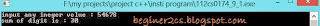## Sunday, 20 October 2013

### Basic question -3 (simple recursion)

This time  we are going to discuss simple recursion .
what is recursion : It is simply a solving technique to solve function which uses its previous volue.
example : Xn = Xn-1 + 2.recursion

It is very  basic example.we can use this technique  in computer science to solve such type of problem very effectivelly . example traversal of tree, find factorial etc.

#### So your Today's challenge is : Make a program to take integer with any no of digits as input & find sum of digits of that integer using  :  2 method

1 => looping
2 => Recursion

example : if integer is 94356 then sum of digits = 9+4+3+5+6 =27.

#### C Program :

Using while loop :

`````` #include<stdio.h>
main()
{
int n,sum=0,p;
printf("input any ineger volue : ");
scanf("%d",&n);
while(n > 0) //
{
p=n%10; // give remainder
sum=sum+p; // count sum
n=n/10; // approx integer of divison i.e 13/10 = 1;
}      // as integer/integer = integer if u wan't in float u need to do typecasting
printf("sum of digit is : %d",sum);
}
``````

#### Using recursion function :

`````` #include<stdio.h>
int sum_of_digits(int,int *); // function declaration
main()
{
int n,sum=0;
printf("input any ineger volue : ");
scanf("%d",&n);
sum_of_digits(n,&sum);
printf("sum of digit is : %d",sum);
}
int sum_of_digits(int a,int *sum)
{
int p;
if(a)  //basicaly it is condition a>0
{    //for a=0 if condition will be false.
p=a%10;
*sum=*sum+p;
sum_of_digits(a/10,sum);
}
return 0;
}
``````

OUTPUT :Sum of digits using recursion & loop